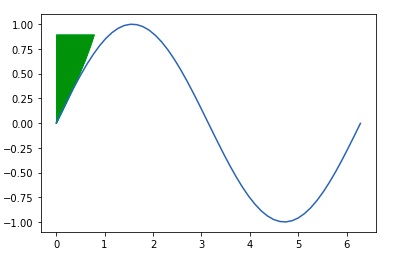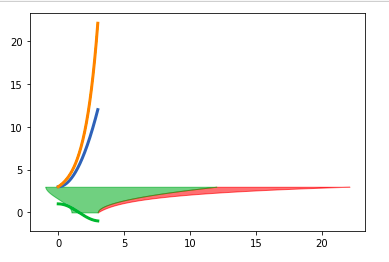# Matplotlib.pyplot.fill_betweenx() in Python

Matplotlib is an amazing visualization library in Python for 2D plots of arrays. Matplotlib is a multi-platform data visualization library built on NumPy arrays and designed to work with the broader SciPy stack.

## matplotlib.pyplot.fill_betweenx()

The matplotlib.pyplot.fill_betweenx() is used to fill area between two vertical curves. Two points (x1, y) and (x2, y) define the curves. this creates one or more polygons describing the filled areas. The ‘where’ parameter can be used to selectively fill some areas. By default, edges connect the given points directly. The ‘step’ parameter is used, if the filling needs to be a step function.

Syntax: matplotlib.pyplot.fill_betweenx(y, x1, x2=0, where=None, step=None, interpolate=False, *, data=None, **kwargs)

Parameters:

• y: It is array of length N. These are the y coordinates of the nodes that define the curves.
• x1:It is an array of length N or a scalar. This represents the x coordinates of the nodes that define the first curve.
• x2: It is an array of length N and is optional in nature. Its default value is 0. This represents the x coordinates of the nodes that define the second curve.
• where: it is an array of boolean values of length N. It is defined if there is a need to exclude some vertical regions from being filled. It is important to note that this definition means that an isolated true value in between two two false values is where it will not do the filling. Adjacent False values results in not filling both sides of the True value.
• interpolate: It is an optional parameter that accepts boolean values. It is only relevant if where is used and two curves are crossing each other. Semantically where if generally used for x1>x2 or similar cases. By default the filled regions will be placed at the y-array positions defining a filled polygonal area. The section of y that has the intersection are simply clipped. Setting this parameter to True results in calculation of the actual point of intersection and extends to the filled regions till the points.
• step: This is an optional parameter that accepts one of the three values namely, ‘pre’, ‘post’ and ‘mid’. This is used to specify where the steps will occur.
• pre: From every x position the y value is continued constantlyto the left, ie, the interval (x[i-1], x[i]) has the value y[i].
• post:From every x position the y value is continued constantly to the right, ie, the interval (x[i], x[i+1]) has the value y[i].
• mid: Half way between the x positions these steps occur.

Returns: It returns a plotted polygon from the PolyCollection.

other Parameters: **kwargs contains keywords from PolyCollection that controls the polygon properties;

Property Description
agg_filter a filter function that takes a (m, n, 3) float array and a dpi value that returns a (m, n, 3) array
alpha float or None
animated bool
array ndarray
capstyle {‘butt’, ’round’, ‘projecting’}
clim a length 2 sequence of floats; may be overridden in methods that have vmin and vmax kwargs.
cmap colormap or registered colormap
antialiased or aa or antialiaseds bool or sequence of bools
clip_box Bbox
clip_on bool
clip_path [(Path, Transform)|Patch|None]
color color or sequence of rgba tuples
contains callable
edgecolor or ec or edgecolors color or sequence of colors or ‘face’
facecolor or fc or facecolors color or sequence of colors
figure figure
gid str
hatch {‘/’, ‘\’, ‘|’, ‘-‘, ‘+’, ‘x’, ‘o’, ‘O’, ‘.’, ‘*’}
in_layout bool
joinstyle {‘miter’, ’round’, ‘bevel’}
linestyle or ls or linestyles or dashes {‘-‘, ‘–‘, ‘-.’, ‘:’, ”, (offset, on-off-seq), …}
linewidth or linewidths or lw float or sequence of floats
norm Normalize
offset_position {‘screen’, ‘data’}
offsets float or sequence of floats
path_effects AbstractPathEffect
picker None or bool or float or callable
path_effects AbstractPathEffect
picker float or callable[[Artist, Event], Tuple[bool, dict]]
rasterized bool or None
sketch_params (scale: float, length: float, randomness: float)
snap bool or None
transform matplotlib.transforms.Transform
url str
urls List[str] or None
visible bool
xdata 1D array
zorder float

Example 1:

 `import` `matplotlib.pyplot as plt ` `import` `numpy as np ` ` `  `a ``=` `np.linspace(``0``,``2``*``3.14``,``50``) ` `b ``=` `np.sin(a) ` ` `  `plt.fill_betweenx(a, b, ``0``, ` `                  ``where ``=` `(a > ``-``0.5``) & (a <``=` `1``), ` `                  ``color``=``'g'``) ` ` `  `plt.plot(a, b) `

Output:Example 2:

 `import` `pylab as plt ` `import` `numpy as np ` ` `  `N  ``=` `np.linspace(``0``,``3``,``200``) ` `A1 ``=` `N``*``*``2` `+` `3` `A2 ``=` `np.exp(N) ``+` `2` `A3 ``=` `np.cos(N) ` ` `  `plt.plot(N, A1, lw ``=` `3``) ` `plt.plot(N, A2, lw ``=` `3``) ` `plt.plot(N, A3, lw ``=` `3``) ` ` `  `plt.fill_betweenx(N, A1, A2,  ` `                  ``color ``=` `'r'``,  ` `                  ``alpha ``=` `.``5``) ` `plt.fill_betweenx(N, A1, A3,  ` `                  ``color ``=` `'g'``, ` `                  ``alpha ``=` `.``5``) ` ` `  `plt.show() `

Outout:My Personal Notes arrow_drop_upCheck out this Author's contributed articles.

If you like GeeksforGeeks and would like to contribute, you can also write an article using contribute.geeksforgeeks.org or mail your article to contribute@geeksforgeeks.org. See your article appearing on the GeeksforGeeks main page and help other Geeks.

Please Improve this article if you find anything incorrect by clicking on the "Improve Article" button below.

Article Tags :

Be the First to upvote.

Please write to us at contribute@geeksforgeeks.org to report any issue with the above content.